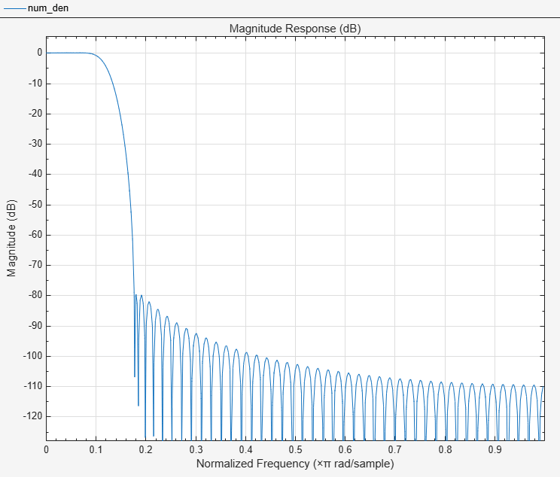# tf

Return transfer function of overall prototype lowpass filter

## Syntax

``[num,den] = tf(obj)``

## Description

example

````[num,den] = tf(obj)` returns the vector of numerator coefficients, `num`, and the vector of denominator coefficients, `den`, for the overall prototype lowpass filter used for the filter bank in `dsp.Channelizer` and `dsp.ChannelSynthesizer` System objects.```

## Examples

collapse all

Determine the transfer function of the overall prototype lowpass filter used for the filter bank in the `dsp.Channelizer` System object.

Design a channelizer with the number of frequency bands or polyphase branches set to 8, the number of taps or coefficients per band set to 12, and stopband attenuation set to 80 dB. The function `tf` returns the transfer function of the prototype lowpass filter.

```channelizer = dsp.Channelizer; [num,den] = tf(channelizer);```

View the magnitude response of the prototype lowpass filter using `fvtool`.

`fvtool(num,den);`## Input Arguments

collapse all

Input filter, specified as either a `dsp.Channelizer` or a `dsp.ChannelSynthesizer` System object.

Example: channelizer = dsp.Channelizer;

Example: ```channelizer = dsp.ChannelSynthesizer```

## Output Arguments

collapse all

Numerator coefficients, returned as a row vector.

Denominator coefficients, returned as a row vector. For a finite Impulse response (FIR) filter, this value is 1.

## Version History

Introduced in R2016b